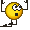# Entropy of a System of Spin-half Non-interacting Particles

## Homework Statement

A system having ##N## non-degenerate energy eigenstates populated by##N## identical spin-zero particles and ##2N## identical spin-half particles. There are no interaction between any of these particles. If ##N=1000## what is the entropy of the system?

## Homework Equations

Third law of thermodynamics $$S=k_B \ln W$$
where ##S## is entropy, ##k_B## is the Botlzmann constant and ##W## is the thermodynamic probability.

Thermodynamic probability, i.e., the total number of independent ways of distributing ##N_i## particles in ##g_i## states is
for ##spin-integral## particles $$W= \prod_{i=1}^{n} {\frac {(N_i +g_i -1)!}{{N_i}! {(g_i -1)!}}}$$
for ##spin-half## particles $$W= \prod_{i=1}^{n} {\frac {{g_i}!}{{N_i}! {(g_i -N_i)!}}}$$

Stirling's formula $$\ln {n!} = n \ln n - n$$

## The Attempt at a Solution

for ##spin-integral## particles $$W={\frac {(N+N -1)!}{{N}! {(N -1)!}}}$$
Simplifying $$W={\frac {(2N -1)!}{{N}! {N!}}}$$
That is approximately $$W={\frac {(2N)!} {{{N}!}^2}}$$
Thus $$S_{spin-0}=k_B \ln{\frac {(2N)!} {{(N!)}^2}}$$
$$\Rightarrow S_{spin-0}=k_B {[\ln {{(2N)!}-\ln {(N!)}^2}]}$$
$$\Rightarrow S_{spin-0}=k_B {[(2N) \ln {(2N)}-(2N)-2\ln {{N}!}]}$$
$$\Rightarrow S_{spin-0}=k_B {[(2N) \ln {(2N)}-(2N)-2N\ln {N} + 2N]}$$
$$\Rightarrow S_{spin-0}=k_B {(2N)[ \ln {(2N)}-\ln {N}]}$$
$$\Rightarrow S_{spin-0}=k_B {(2N) \ln 2}$$

for ##spin-half## particles $$W={\frac {N!}{{(2N)}! {(N -2N)!}}}$$
Which gives a ##negative## factorial at the denominator!
So, does this mean one cannot distribute ##2N## spin-half particles in ##N## states?
Which sounds right, because of the Pauli exclusion principle.

Anyone have any thought?

Related Advanced Physics Homework Help News on Phys.org
PeterDonis
Mentor
2019 Award
Moderator's note: Moved to advanced physics homework forum.

•VSayantan
Is it possible that since a given energy state can accommodate two spin-half particles, at most, there is only one to distribute these ##2N## particles in ##N## states?

PeterDonis
Mentor
2019 Award
Is it possible that since a given energy state can accommodate two spin-half particles, at most, there is only one to distribute these ##2N## particles in ##N## states?
Yes, that is indeed possible, since it's the correct answer.Now you need to consider the case of bosons (spin-integral particles): unlike fermions, multiple bosons can be in the same energy state, and in fact they are more likely to be in the same energy state. What does that do to the probability?

•VSayantan
Yes, that is indeed possible, since it's the correct answer.Now you need to consider the case of bosons (spin-integral particles): unlike fermions, multiple bosons can be in the same energy state, and in fact they are more likely to be in the same energy state. What does that do to the probability?
For the bosons I've already calculated the entropy.

PeterDonis
Mentor
2019 Award
For the bosons I've already calculated the entropy.
In your calculation, you appeared to assume that the bosons had an equal probability of being in any of the ##N## states. But since the states are non-degenerate, one of them must have the lowest energy, and one would expect the ground state of the system to be one in which all of the bosons were in that lowest energy state. There is only one such arrangement.

One thing that's not clear, though, is whether the problem statement specifies a temperature for the system, or expects you to give the entropy as a function of the temperature. At a finite temperature, there will indeed be a nonzero probability of some of the bosons being in one of the energy states other than the lowest energy one.

•VSayantan
There are six options given
##13.82~k_B##
##693.1~k_B##
##zero##
##1000~k_B##
##5909.693~k_B##
##6909~k_B##

And no the question does not ask for entropy as a function of temperature.
Thanks for your suggestion @PeterDonisPeterDonis
Mentor
2019 Award
There are six options given
Yes. Which one do you think is the right answer? Note that you've already shown that, for the fermions alone, the answer is zero (because that corresponds to there being only one way of arranging the particles).

•VSayantan
Yes. Which one do you think is the right answer? Note that you've already shown that, for the fermions alone, the answer is zero (because that corresponds to there being only one way of arranging the particles).
And you argued that that it is most probable for the bosons to occupy the same state. Which gives only one most probable arrangement, when there is no constraint of temperature involved.

So, I think you're right and zero is the correct choice, I think.

PeterDonis
Mentor
2019 Award
I think you're right and zero is the correct choice
Another indication is that none of the choices match the answer you calculated in the OP for the bosons: ##k_B (2N) \ln 2## gives ##(2000) 0.693 k_B = 1386 k_B##.

•VSayantan
Another indication is that none of the choices match the answer you calculated in the OP for the bosons: ##k_B (2N) \ln 2## gives ##(2000) 0.693 k_B = 1386 k_B##.
Yes.
I tried to convince myself it was the closest answer (which would be a 100 times different than ##13.82~k_B##) before you suggested the one state population scenario.